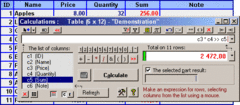Search
Software
Options for SumsBox-W for MS Word
SumsBox-W for MS Word
The add-in "SumsBox" will add new opportunities to the MS Word, speed up mathematical calculations, make your work with numbers and tables easy and pleasant.
The add-in is an original calculator used for calculating in the selected table cells or the entered expression allowing autofilling the fields of tables.
When you open the add-in it automatically calculates the sum of the selected table column. If some columns (rows) are selected it is necessary to make an expression consisting of the names of columns (rows). Then the sum of results of this expression for the selected cells will be calculated.
Results in rows are summed from top to bottom and in columns from left to right.
One can use the add-in to calculate any expression using either the names of selected columns (rows) or without them being included into the expression. One can include into the expression arithmetic and string operators and VBA functions.
The add-in provides autofilling with numbers of the columns (rows) (the values of numbers are either set or calculated) and also autofilling with formulas (by Word fields).
When autofilling is performed one can set conditions in the Filter Window.
It is possible to carry out a series of operations using a serial expression at a time.
Moreover one can insert dates, numbers, a selected fragment in the text of the table into the expression by pressing the left mouse button one time. To perform this function it is necessary to switch on the Mouse insertion mode or hold down the controlling combination of keys.
Calculations can be made in the calculator mode.